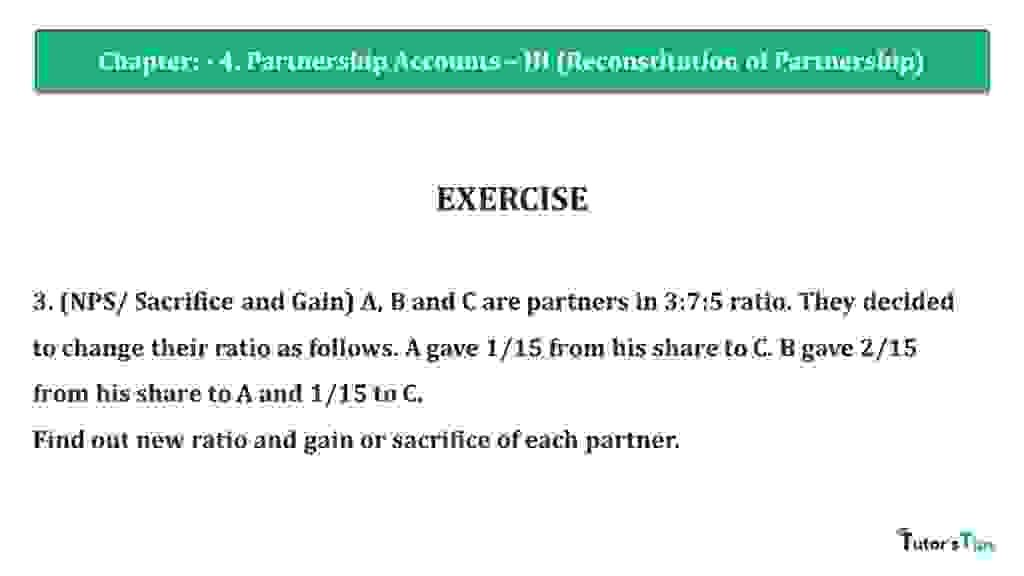# Question 03 Chapter 4 of +2 Part-1 – USHA Publication 12 Class Part – 1Question 03 Chapter 4 of +2-Part-1

3. (NPS/ Sacrifice and Gain) A, B and C are partners in 3:7:5 ratio. They decided to change their ratio as follows. A gave 1/15 from his share to C. B gave 2/15 from his share to A and 1/15 to C.
Find out new ratio and gain or sacrifice of each partner.

### The solution of Question 03 Chapter 4 of +2 Part-1: –

Calculate the A, B, & C New Profit sharing ratio: –

Sacrificing or Gaining Ratio = Old Ratio – New Ratio

 A’s Gain = 2 – 5 – 2 15 15 15
 = 3 – 1 + 2 15
 = 4 15
 B’s New Share = 7 – 2 – 1 15 15 15
 = 7 – 2 – 1 15
 = 4 15
 C’s New Share = 5 + 1 + 1 15 15 15
 = 5 + 1 + 1 15
 = 7 15

New Profit Ratio of A,B, & C = 4 : 4: 7

Calculate the Sacrificing or Gaining Ratio of Partners:
Sacrificing or Gaining Ratio = Old Ratio – New Ratio

 A’s Share Sacrificing/Gaining = 3 – 4 15 15
 = 3 – 4 15
 = -1 (Gain) 14

 B’s Sacrifice = 7 – 4 15 15
 = 7 – 4 15
 = -3 (Sacrifice) 30

 C’s Gain = 5 – 7 15 15
 = 5 – 7 15
 = -2 (Gain) 15

Thanks, Please Like and share with your friends

Comment if you have any questions.

Also, Check out the solved question of previous Chapters: –

## Usha Publication – Accountancy PSEB (Class 12) – Volume II – SolutionVol. I: Accounting for Not-for-Profit Organizations and Partnership Firms